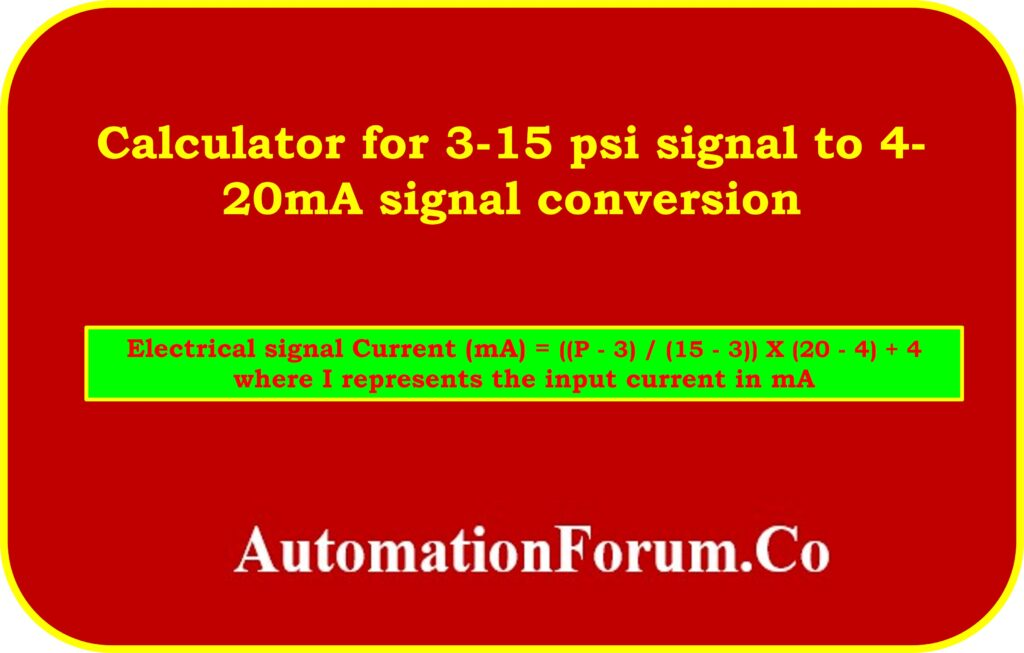# Calculator for 3-15 psi signal to 4- 20mA signal conversionA calculator for converting a standard 3-15 psi signal to a 4-20mA signal is a useful tool in industrial instrumentation and control systems. This conversion is commonly required in process control applications where different types of instruments are used to measure and control variables such as pressure, level, or flow.

The 3-15 psi signal is a widely used range in pneumatic instrumentation, where pressure is used to transmit and control signals. On the other hand, the 4-20mA signal is a common standard in electronic instrumentation due to its ability to transmit analog data accurately and reliably.

## Formula for the signal conversion

To convert the 3-15 psi signal to a 4-20mA signal, a linear conversion can be applied using the following formula:

Current (mA) = ((P – 3) / (15 – 3)) X (20 – 4) + 4

where P represents the input pressure in psi.

This formula calculates the corresponding current value within the 4-20mA range based on the input pressure.

## Break down the formula:

• Subtracting 3 from the input pressure (P – 3) shifts the range from 3-15 psi to 0-12 psi.
• Dividing the shifted pressure value by the range of the input signal (15 – 3) normalizes the value between 0 and 1.
• Multiplying the normalized value by the range of the output signal (20 – 4) scales the value to the desired range.
• Finally, adding the minimum value of the output signal (4) gives the converted current value in mA.

## Example calculation

For example, if the input pressure is 9.21 psi, we can substitute it into the formula:

Current (mA) = ((9.21 – 3) / (15 – 3)) * (20 – 4) + 4

Current (mA) = (6.21 / 12) * 16 + 4

Current (mA) = 0.5175 * 16 + 4

Current (mA) = 8.28 + 4

Current (mA) = 12.28 mA

In this case, a 9.21 psi input pressure corresponds to a 12.28 mA current value.

The calculator simplifies the conversion process by automating the calculation for various input pressure values, allowing engineers and technicians to easily determine the corresponding current values in mA. This conversion enables seamless integration between instruments using different signal types and ensures accurate measurement and control in industrial processes.

## Signal calculator

The following instrumentation calculator will convert standard 3-15 psi signals to 4- 20mA signals.

Scroll to Top